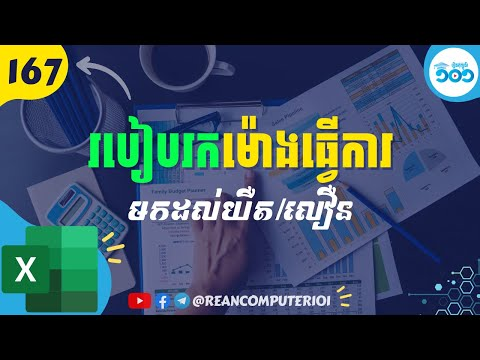## How to calculate early or late for working in Excel with Example Download

This tutorila show you how to calulcate whether you are early or late for working hour in the morning and how many minute or hour of late or early in excel. There are four main formulas in this tutorial.### IF formula in Excel

IF returns value of true_value or false_value base on the condition.
FORMULA:
=IF(logical_test, [value_if_true], [value_if_false])
◾ logical_test: Required, condition to compare.
◾ [value_if_true]: return value if condition is true
◾ [value_if_false]: return value if condition is false

### TEXT formula in Excel

TEXT convert value to text format as you want.
FORMULA:
=TEXT(value, format_text)
◾ value: Required, value you want to format.
◾ value: Required, format you want to apply.

### HOUR formula in Excel

HOUR returns hour value from time or datetime value.
FORMULA:
=HOUR(serial_number)
◾ serial_number: Required, time or date contains hour.

### MINUTE formula in Excel

MINUTE returns minute value from time or datetime value, range from 0 to 59.
FORMULA:
=MINUTE(serial_number)
◾ serial_number: Required, time or date contains hour.
Watch How to caluclate early or late for working hour in Excel speak Khmer